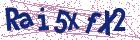# Radial basis functions method for solving three-dimensional linear Fredholm integral equations on the cubic domains

Document Type : Research Article

Authors

Department of Mathematics, Faculty of Mathematical Sciences and Statistics, Malayer University, Malayer, Iran.

Abstract

The main purpose of this article is to describe a numerical scheme for solving three-dimensional linear Fredholm integral equations of the second kind on the cubic domains. The method is based on interpolation by radial basis functions (RBFs) based on Gauss-Legendre nodes and weights. Error analysis is presented for this method. Finally, several examples are given and numerical examples are presented to demonstrate the validity and applicability of the method.

Keywords20.1001.1.24236977.2017.7.2.2.3

#### References

 Guoqiang, H. and Jiong, W. Extrapolation of nystrom solution for two dimensional nonlinear Fredholm integral equations, J. Comput. Appl. Math.134 (2001), 259-268.
 Guoqiang, H., Hayami, K., Sugihara,K. and Jiong, W. Extrapolation method of iterated collocation solution for two-dimensional nonlinear Volterra integral equations, Appl. Math. Comput. 112 (2000), 49-61.
 Brunner, H. Collocation Methods for Volterra Integral and Related Functional Equations, Cambridge University Press, 2004.
 Xie, W. J. and Lin, F. R. A fast numerical solution method for two dimensional Fredholm integral equations of the second kind, Applied Numerical Mathematics, 59(2009), 1709-1719.
 Salehi, R. and Dehghan, M. A moving least square reproducing polynomial meshless method, Appl. Numer. Math. 69 (2013), 34-58.
 Dehghan, M. and Salehi, R. The numerical solution of the non-linear integro-differential equations based on the meshless method, J. Comput. Appl. Math. 236, 2367-2377 (2012)
 Dehghan, M. and Mirzaei, D. Meshless Local Petrov-Galerkin (MLPG) method for the unsteady magnetohydrodynamic (MHD) flow through pipe with arbitrary wall conductivity, App. Numer. Math. 59 (2009), 1043-1058.
 Kansa, E.J. Multiquadrics - A scattered data approximation scheme with applications to computational fluid dynamics-I, Comput. Math. Appl. 19(1990) 127-145.
 Kansa, E.J. Multiquadrics - A scattered data approximation scheme with applications to computational fluid dynamics-II, Comput. Math. Appl. 19(1990) 147-161.
 Hon, Y.C. and Mao, X.Z. An efficient numerical scheme for Burgers equation, Appl. Math. Comput. 95 (1) (1998) 37-50.
 Hon, Y.C., Cheung, K.F., Mao, X.Z. and Kansa, E.J. Multiquadric solution for shallow water equations, ASCE J. Hydraul. Eng. 125 (5)
(1999) 524-533.
 Zerroukat, M., Power, H. and Chen, C.S. A numerical method for heat transfer problem using collocation and radial basis functions, Internat. J. Numer. Methods Engrg. 42 (1992) 1263-1278.
 Hon, Y.C. and Mao, X.Z. A radial basis function method for solving options pricing model, Financ. Eng. 8 (1) (1999) 31-49.
 Marcozzi, M., Choi, S. and Chen, C.S. On the use of boundary conditions for variational formulations arising in financial mathematics, Appl. Math. Comput. 124 (2001) 197-214.
 Golbabai, A. and Seifollahi, S. Numerical solution of the second kind integral equations using radial basis function networks, Appl. Math. Comput. 174 (2006) 877-883.
 Golbabai, A. and Seifollahi, S. Radial basis function networks in the numerical solution of linear integro-differential equations, Appl. Math. Comput. 188 (2007) 427-432.
 Parand, K. and Rad, J.A. Numerical solution of nonlinear Volterra Fredholm-Hammerstein integral equations via collocation method based on radial basis functions, Appl. Math. Comput. 218 (2012) 5292-5309.
 Golbabai, A., Mammadov, M. and Seifollahi, S. Solving a system of nonlinear integral equations by an RBF network, Comput. Math. Appl. 57 (2009) 1651-1658.
 Alipanah, A. and Esmaeili, S. Numerical solution of the two-dimensional Fredholm integral equations using Gaussian radial basis function, J. Comput. Appl. Math. 235 (2011) 5342-5347.
 Hardy, R. L. Multiquadric equations of topography and other irregular surfaces, J. Geophys. Res. 176 (1971) 1905-1915.
 Franke, R. Scattered data interpolation: Tests of some methods, Mathematics of Computation, 38(1982) 181-200.
 Wendland, H. Scattered data approximation, Cambridge Monographs on Applied and Computational Mathematics, vol. 17. Cambridge University Press, Cambridge (2005).
 Fasshauer, G. E. Meshfree approximation methods with MATLAB, Interdisciplinary Mathematical Sciences, vol. 6. World Scientific Publishing Company, Singapore (2007).
 Schoenberg, I. J. Metric spaces and completely monotone functions, Ann. Math., 39: 811-841, 1938.
 Micchelli, C. A. Interpolation of scattered data: distance matrices and conditionally positive denite functions, Constr. Approx., 2: 11-22, 1986.
 Larsson, E. and Fornberg, B. A numerical study of some radial basis function based solution methods for elliptic PDEs, Comput. Math. Appl. 46 (2003) 891-902.
 Assari, P., Adibi, H. and Dehghan, M. A numerical method for solving linear integral equations of the second kind on the non-rectangular domains based on the meshless method, Applied Mathematical Modelling, vol. 37, pp. 9269-9294, 2013.
 Fasshauer, G. E. Newton iteration with multiquadrics for the solution of nonlinear PDEs, Computers and Mathematics with Applications, 43: 423-438, 2002.
 Atkinson, K. E. and Han, W. Theoretical Numerical Analysis: a Func tional Analysis Framework, Springer-Verlag New York, INC, 2001.
 Atkinson, K. E. The Numerical Solution of Integral Equations of the Second Kind, vol. 4, Cambridge University Press, Cambridge, UK, 1997.